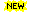## Chih-Jen Lin

### IntroductionThe code can be directly run on GPU. See example below. (added on April 19, 2020)

This tool solves NMF by alternative non-negative least squares using projected gradients. It converges faster than the popular multiplicative update approach. Details and comparisons are in the following paper:

C.-J. Lin. Projected gradient methods for non-negative matrix factorization. Neural Computation, 19(2007), 2756-2779.

If you find this tool useful, please cite the above work.

[W,H] = nmf(V,Winit,Hinit,tol,timelimit,maxiter);

Output/input parameters are

• W,H: output solution
• Winit,Hinit: initial solution
• tol: stopping tolerance
• timelimit, maxiter: limit of time and iterations

This code works under Octave as well.

### Running on GPU

The code can be directly used without modification, but input matrices must be MATLAB gpuArray. See a simple example here

### Sources for comparing different methods for solving NMF

We compared our approach with the popular multiplicative update method in the above paper. All sources for experiments are in this zip file. The current version is 1.01. You can thus easily regenerate our results. After unzipping it, a directory including all files will be created. Then follow instructions in the README file.

Note that some sources here cannot be used under Octave.